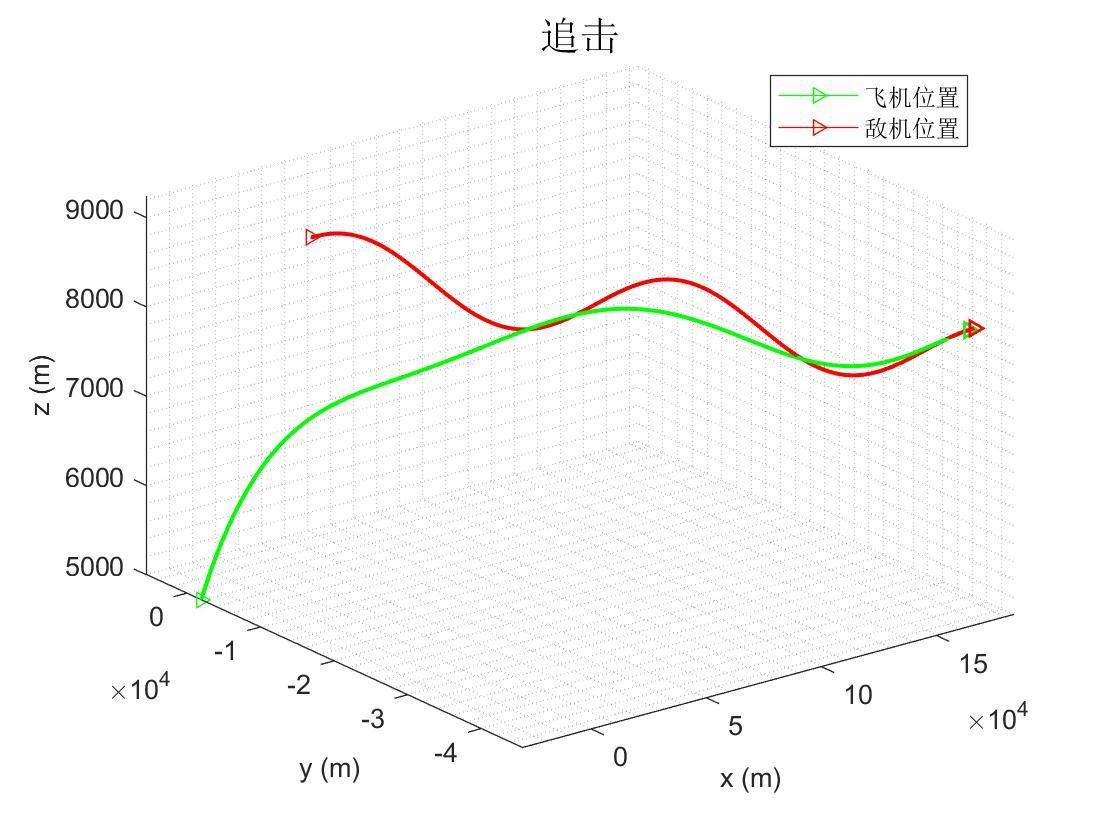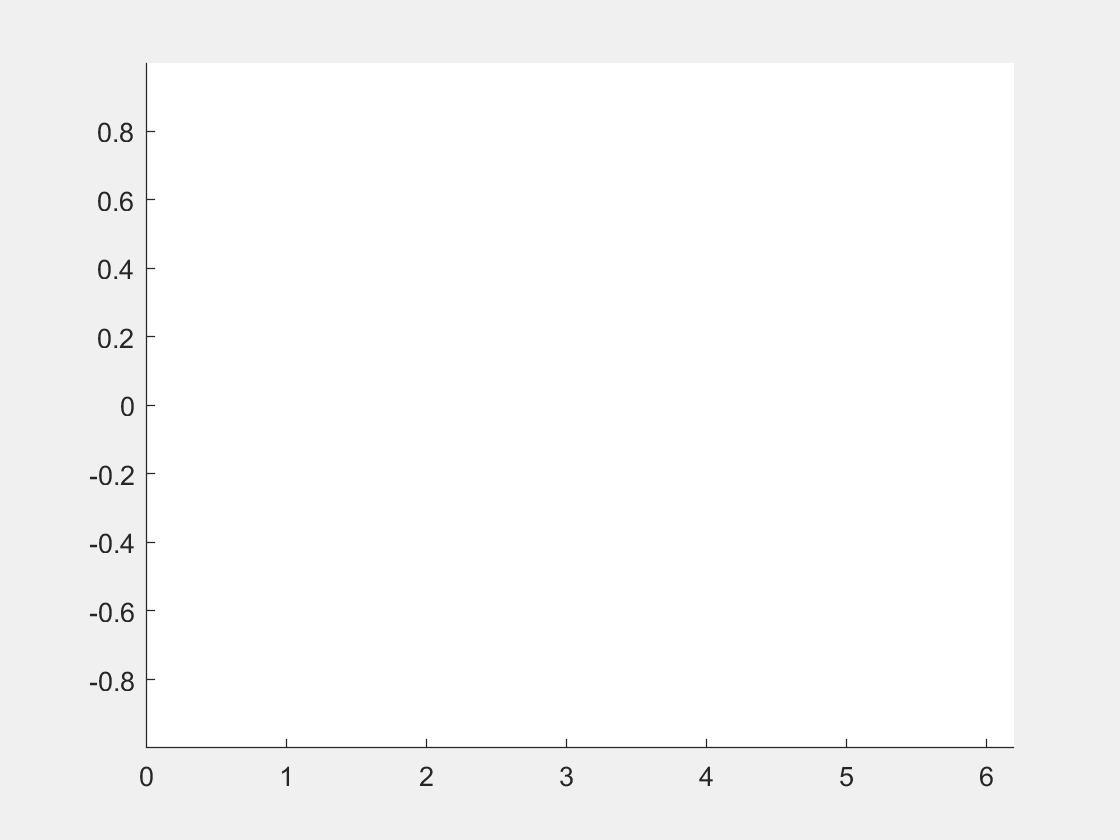# 保存plot_MATLAB保存动态图## 1.动态曲线例：x = 0:0.1:2*pi;
y = sin(x);
axis([min(x) max(x) min(y) max(y)]);
line = animatedline('Color','r','LineWidth',3);
for i=1:length(x)
addpoints(line,x(i),y(i));
pause(0.1);
drawnow;
end
1. line是animatedline函数返回的一个结构体（和C语言意思差不多）变量，包含曲线各种属性，后续也可以修改。
2. axis主要是用来对坐标轴进行一定的缩放操作，其操作命令主要如下：
• axis( [xmin xmax ymin ymax zmin zmax] ) %设置 x,y,z轴的限制范围。

3.addpoints(line,x(i),y(i)),向已有曲线添加点。并且drawnow配和使用，表示画出新曲线。

4.为限制过快更新，以至于看不到效果，使用pause（0.1）暂停程序0.1s## 2.介绍存介绍一个存图子程序：

MakeGif(filename,index)

• filename为保存图像文件名，注意单引号，
• index表示迭代索引。
• 按照 一定时间间隔顺序保存 当前MATLAB figure窗口图像：（由于figure窗口图片在变化，我们保存的就是一系列变化的图片序列，再按顺序播放形成动态图）
function MakeGif(filename,index)
f = getframe(gcf);
imind = frame2im(f);
[imind,cm] = rgb2ind(imind,256);
if index==1
imwrite(imind,cm,filename,'gif', 'Loopcount',inf,'DelayTime',0.001);
else
imwrite(imind,cm,filename,'gif','WriteMode','append','DelayTime',0.001);
end
end  

• 指定 0，动画将播放一次。
• 指定 1，动画将播放两次，以此类推。
• 指定Inf值可使动画连续循环。

x = 0:0.1:2*pi;
y = sin(x);
axis([min(x) max(x) min(y) max(y)]);
line = animatedline('Color','r','LineWidth',3);
for i=1:length(x)
addpoints(line,x(i),y(i));
MakeGif('my.Gif',i);
pause(0.1);
drawnow;
end

## 3.动态曲面08-10
01-031万+
11-254万+
08-161940
11-277168
07-101万+
04-14888
12-23850
12-29716
©️2020 CSDN 皮肤主题: 1024 设计师:白松林